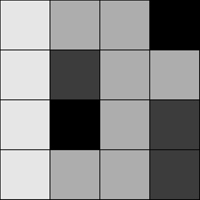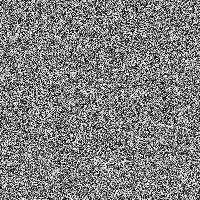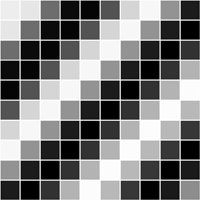##帐号 自动登录 找回密码 密码 注册
 搜索

# Processing官网学习部分原文翻译——二维数组发表于 2012-11-10 22:34:17 | 显示全部楼层 |阅读模式
 本帖最后由 弘毅 于 2012-11-19 18:14 编辑 二维数组 本手册适用于Processing1.1及以上版本。如果你发现任何错误或者有任何建议，请联系我们。本手册源自《学习Processing》一书，Daniel Shiffman撰写，Morgan Kaufmann出版社出版，Elsevier公司2008版权，版权所有。 一个数组用一个一维列表线性顺序地联系着多个信息模块。然而，某些系统（一个数字图像，一个棋盘游戏等）的相关数据存在于两个维度。为了形象化这些数据，我们需要一个多维数据结构，即一个多维数组。 两位数组无非就是一个数组的数组（三维数组就是数组的数组的数组PS晕了~）。想想你的晚餐，你有一个你吃的任何东西的一维列表： （生菜，番茄，沙拉，牛排，土豆泥，豆角，蛋糕，冰淇淋，咖啡） 或者你可以有一个包含三个课程的两位数组列表，每个都包含三个你吃的东西： （生菜，番茄，沙拉）和（牛排，土豆泥，豆角）和（蛋糕，冰淇淋，咖啡） 对于一个数组，我们旧式的一维数组看起来像是这样： [pre lang="processing" line="1"]int myArray = {0,1,2,3};[/code] 二维数组像这样： [pre lang="processing" line="1"]int myArray = { {0,1,2,3}, {3,2,1,0}, {3,5,6,1}, {3,8,3,4} };     [/code] 根据我们的目标，最好把二维数组看做一个矩阵。矩阵可视为按照行和列来的网格数据，有点像宾戈游戏板。 我们可以写个像下面的二维数组来说明这点 [pre lang="processing" line="1"]int myArray = {  {0, 1, 2, 3},                   {3, 2, 1, 0},                   {3, 5, 6, 1},                   {3, 8, 3, 4}  };[/code] 我们可以使用这种类型的数据结构来编码图像信息。例如，下面的灰度图像可以通过以下数组代替：:[pre lang="processing" line="1"]int myArray = {  {236, 189, 189,   0},                   {236,  80, 189, 189},                   {236,   0, 189,  80},                   {236, 189, 189,  80}  };[/code] 为了贯穿一维数组的所有元素，我们使用一个for循环，即： [pre lang="processing" line="1"]int myArray = new int; for (int i = 0; i < myArray.length; i++) {   myArray = 0; }[/code] 对于一个二维数组，为了引用每个元素，我们得使用两个嵌套循环。这儿为矩阵的每行每列给了一个变量计算器。 [pre lang="processing" line="1"]int cols = 10; int rows = 10; int myArray = new int[cols][rows]; //两个嵌套循环允许我们访问二维数组的每一个点。 // 对每一列I,，访问每一行J. for (int i = 0; i < cols; i++) {   for (int j = 0; j < rows; j++) {     myArray[j] = 0;   } }[/code] 例如，我们用二维数组编程画一个灰度图。[pre lang="processing" line="1"]// 例子：二维数组 size(200,200); int cols = width; int rows = height; //声明二维数组 int myArray = new int[cols][rows]; // 初始化二维数组变量 for (int i = 0; i < cols; i++) {   for (int j = 0; j < rows; j++) {     myArray[j] = int(random(255));   } } //画点 for (int i = 0; i < cols; i++) {   for (int j = 0; j < rows; j++) {     stroke(myArray[j]);     point(i,j);   } }[/code] 二维数组同样可以用来储存对象，这个对于对包含一些“网格”或者“板”的草图编程尤为方便。下面啊的例子显示了储存在二维数组中的单元格对象网格。每个单元格是一个亮度以正弦函数从0到255震荡变动。例子：二维对象数组 [pre lang="processing" line="1"]//二维的对象数组 Cell grid; // 网格行、列数 int cols = 10; int rows = 10; void setup() {   size(200,200);   grid = new Cell[cols][rows];   for (int i = 0; i < cols; i++) {     for (int j = 0; j < rows; j++) {       // Initialize each object       grid[j] = new Cell(i*20,j*20,20,20,i+j);     }   } } void draw() {   background(0);   // 计数器变量i和j同样是行、列数，并且   //在网格中作为每个对象构造函数的参数使用   for (int i = 0; i < cols; i++) {     for (int j = 0; j < rows; j++) {       // 震动变化并显示每个对象       grid[j].oscillate();       grid[j].display();     }   } } // A Cell object class Cell {   //通过变量x、y、w、h能够知道一个单元格在网格中的位置以及大小。   float x,y;   // x轴、y轴位置   float w,h;   //宽和高   float angle; // 获取震荡亮度   // 单元构造函数   Cell(float tempX, float tempY, float tempW, float tempH, float tempAngle) {     x = tempX;     y = tempY;     w = tempW;     h = tempH;     angle = tempAngle;   }      //震荡意味着增加函数   void oscillate() {     angle += 0.02;   }   void display() {     stroke(255);     // 使用正弦波计算颜色     fill(127+127*sin(angle));     rect(x,y,w,h);   } }[/code]发表于 2014-12-1 14:30:45 | 显示全部楼层
 原文翻译系列对于我们这些刚开始学习的学生来说真的是受益匪浅，灰常感谢楼主～～～～～～发表于 2014-12-1 14:45:07 | 显示全部楼层
 不过二维对象数组哪个案例怎么复制粘贴运行有错误？

 本版积分规则 回帖后跳转到最后一页

GMT+8, 2020-2-21 21:02 , Processed in 0.053016 second(s), 27 queries .